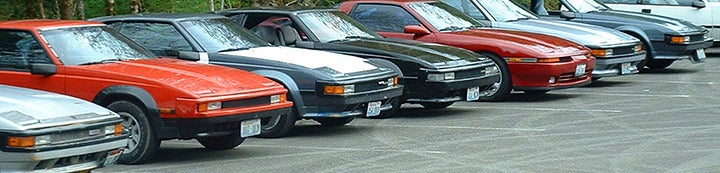1 - 6 of 6 Posts
G

#### Guest

·
Discussion Starter · ·
i am looking for a rear diff for my 350 v8 supra it needs to be a 3.70 or higher. i am currently running a 4.10 and its way too stiff. open diff out of a l type would be preferred. pm me or call my cell 1-540-454-6594 my name is Brandon and i'm located in northern Virginia 20135. if you could get any shipping prices. thanks

#### zuprax85

·
##### Registered
Joined
·
3,226 Posts
i can get you one off an 82, i guess is a 3.73, not quite sure, i can get it for you this weekend. pm me if you are interested.

#### Dave_Cod

·
##### Registered
'84 Dark Blue Converted to 5 Spd... '85 ..gradually acquiring parts to Turbo ... have 82 Engine
Joined
·
1,451 Posts
If he's not interested, I might be if we can determine it's a 3:73 for sure...do U still have the car that the diff is out of??? There's a way of telling by the VIN plate what the gear ratio is...

#### supra_toy

·
##### Registered
Joined
·
2,134 Posts

First Digit
(Ring Gear Diameter)
A = 138 mm
B = 145 mm
C = 6.25 "
D = 6.62"
E = 7.1"
F = 7.5"
G = 8"
H = 9"
J = 9.25"
K = 9.5"
L = 10.5"
M = 12.5"
N = 13.5"
P = 14"
R = 162 mm
S = 6.38"
T = 6.7"
U = 6"
V = 10.6"
W = 15.5"
X = 142 mm
Y = 158 mm

Second & Third Digits
(Gear Ratio)
01 = 3.30
02 = 3.36
03 = 3.545
04 = 3.556
05 = 3.70
06 = 3.889
07 = 3.90
08 = 4.111
09 = 4.222
10 = 4.375
11 = 4.444
12 = 4.625
13 = 4.79
14 = 4.875
15 = 5.125
16 = 5.286
17 = 5.60
18 = 5.714
19 = 5.833
20 = 6.167
21 = 6.667
22 = 6.78
23 = 6.833
24 = 7.64
25 = 4.556
26 = 5.571
27 = 3.364
28 = 4.30
29 = 4.10
30 = 3.727
31 = 3.909
32 = 6.591 or 5.583
33 = 7.503 or 5.583
34 = 6.781 or 4.786
35 = 7.636 or 5.60
36 = 4.778
37 = 3.583
38 = 3.417

Fourth Digit
(# of Pinion Gears Limited Slip (yes/no)
2 = 2 Pinions/No LSD
3 = 2 Pinions /Yes LSD
4 = 4 Pinions/No LSD
5 = 4 Pinions/Yes LSD

G

#### Guest

·
Discussion Starter · ·
thats awesome thanks alot

#### rabidchimp.com

·
##### Registered
Joined
·
3,291 Posts
I can provide one for you. \$300 shipped.
-Aaron

1 - 6 of 6 Posts